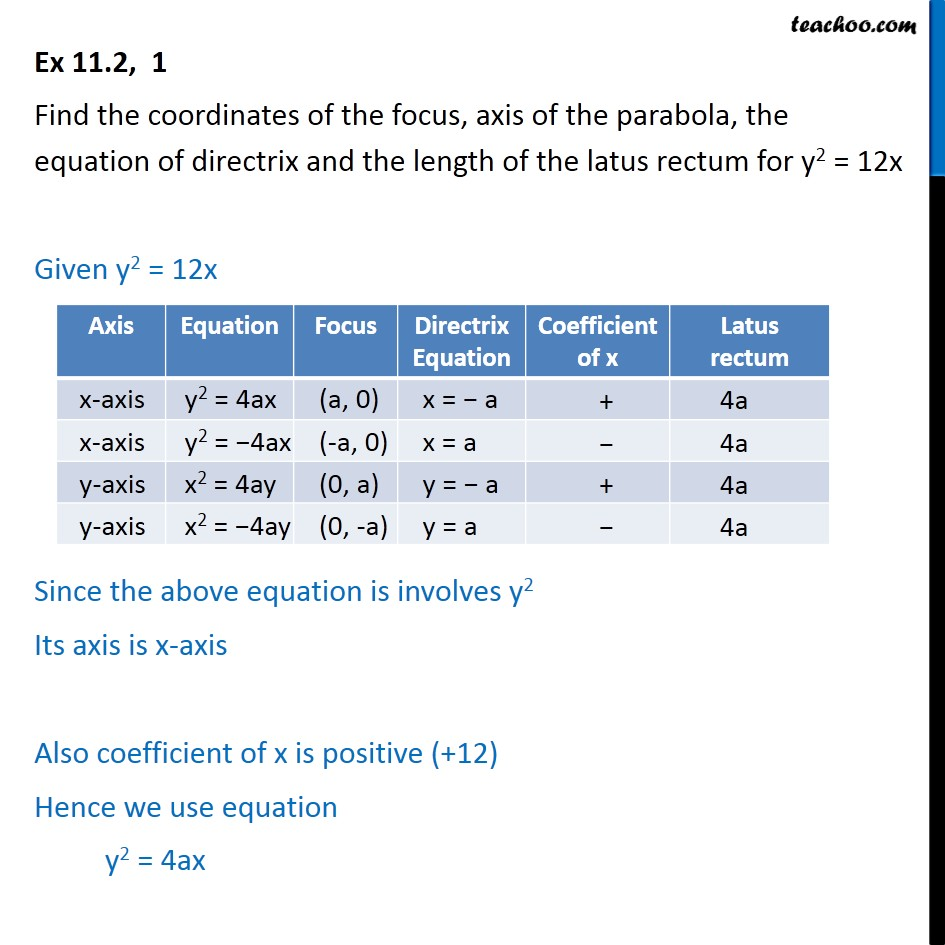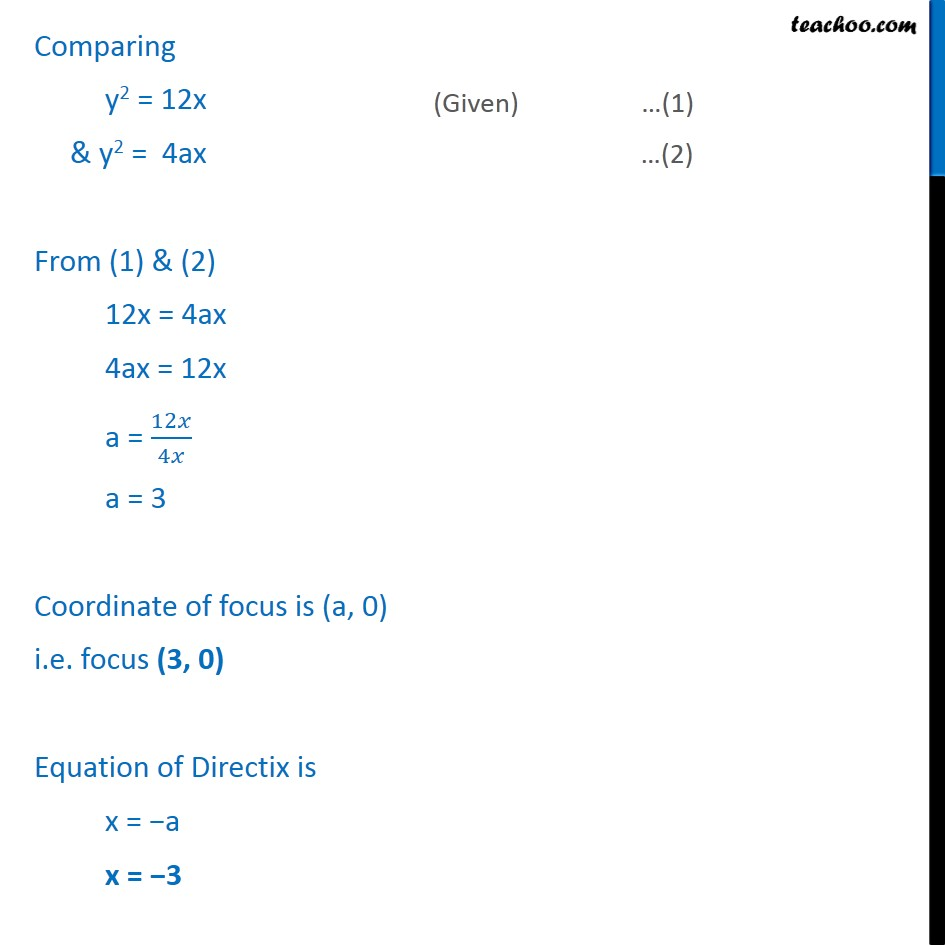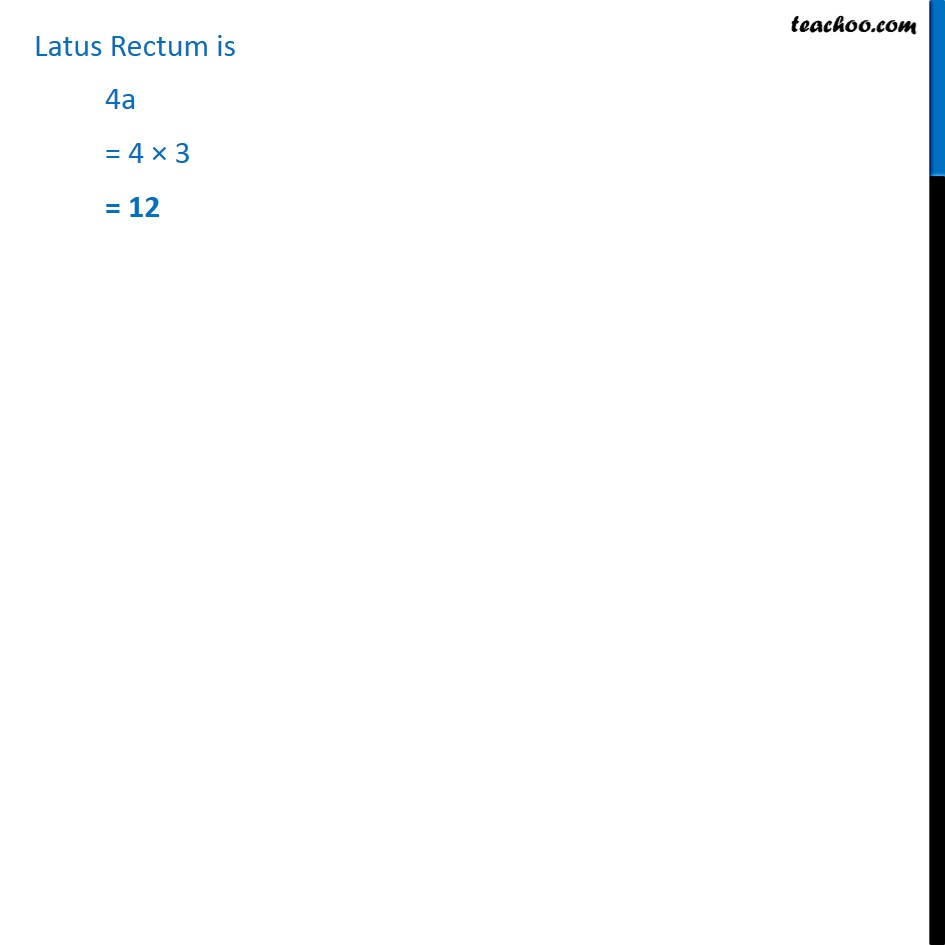Ex 10.2

Chapter 10 Class 11 Conic Sections
Serial order wiseLearn in your speed, with individual attention - Teachoo Maths 1-on-1 Class

### Transcript

Ex 10.2, 1 Find the coordinates of the focus, axis of the parabola, the equation of directrix and the length of the latus rectum for y2 = 12x Given y2 = 12x Since the above equation is involves y2 Its axis is x-axis Also coefficient of x is positive (+12) Hence we use equation y2 = 4ax Latus Rectum is 4a = 4 × 3 = 12